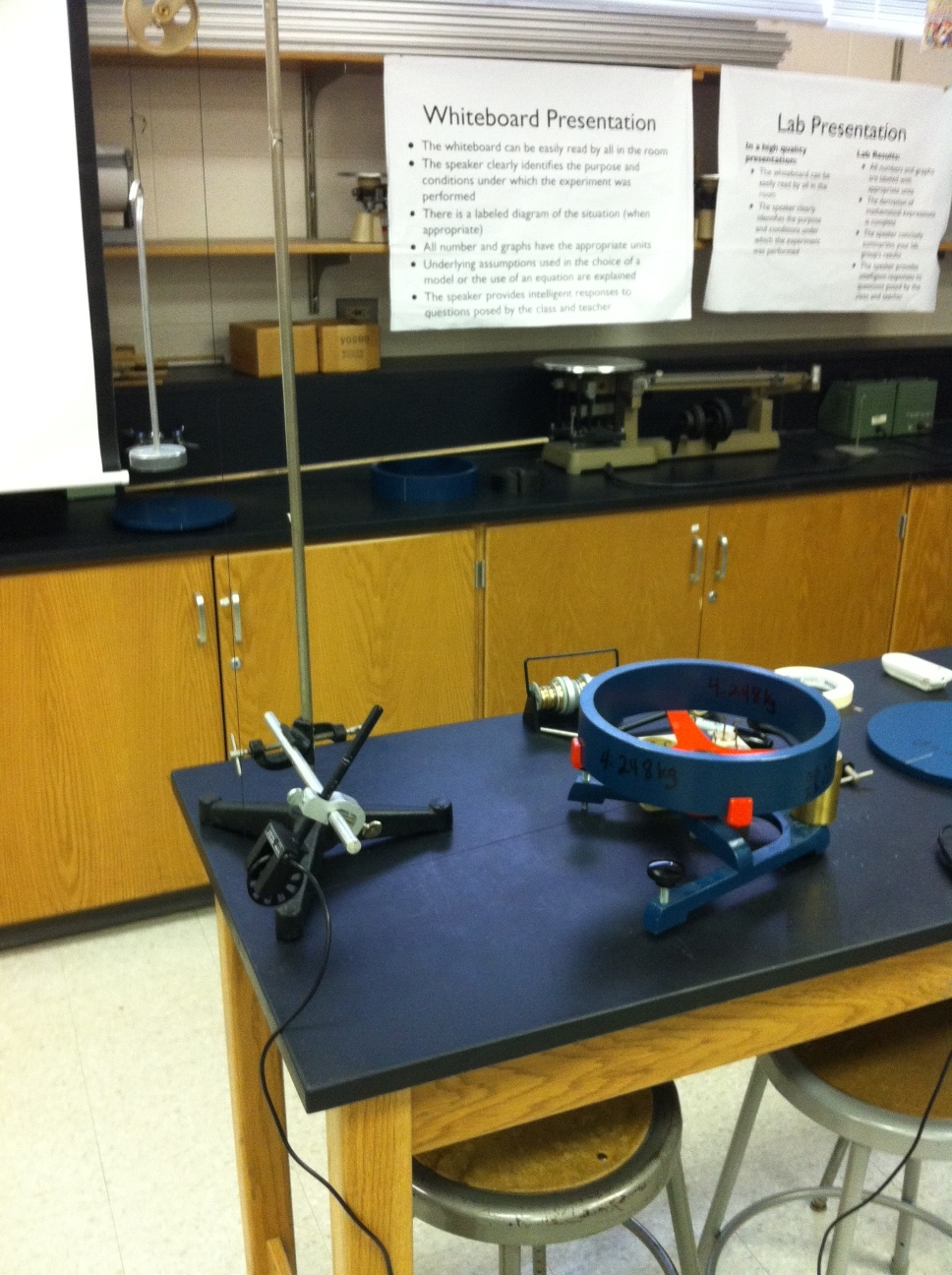# Day 113: Moment of Inertia Lab

Today’s lab we are doing as a whole class in more of an interactive lecture demonstration format. The apparatus, procedure, and analysis are somewhat involved and I want to ensure that each student understands each step along the way. The purpose is to experimentally determine the moment of inertia of a solid disc and a ring. We will do this by first determining the angular acceleration of the system by graphing angular velocity vs. time. We will then determine the moment of inertia of the system by graphing applied torque vs. angular acceleration. We have to first determine the moment of the apparatus so we can subtract that from the moment of the disc and ring. There is a great opportunity for a side discussion of how the vertical intercept of the torque vs. angular acceleration graph is the torque due to friction in the bearing.

The data we collected in class looked good, but the experimental results show a moment of inertia ten times greater than what is calculated using the mass and geometry of the disc. We haven’t figured out what the problem is. We did realize that we weren’t accounting for the acceleration of the mass hanger and, therefore, the tension in the string is less than the force of gravity. However, I fixed that, and it had minimal impact on the results. If anyone sees a flaw in the experimental design, please let me know!